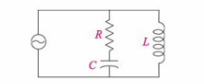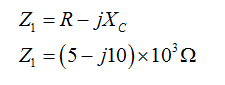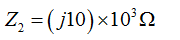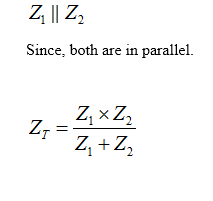Question
1 views

Find ZT.

R=5kohms, XC=10kohms, Xl=10kohms

check_circle

analysis of the circuit

the given circuit has two branches that are connected in parallel, in the first branch the capacitor and the inductor are connected in series and in other there is inductance.

the circuit is shown below:calculation of the impedance of each branches

calculation of the impedance of each branch. The impedance  is given as:The impedance  is given as:The overall impedance is given as:...

### Want to see the full answer?

See Solution

#### Want to see this answer and more?

Solutions are written by subject experts who are available 24/7. Questions are typically answered within 1 hour.*

See Solution
*Response times may vary by subject and question.
Tagged in